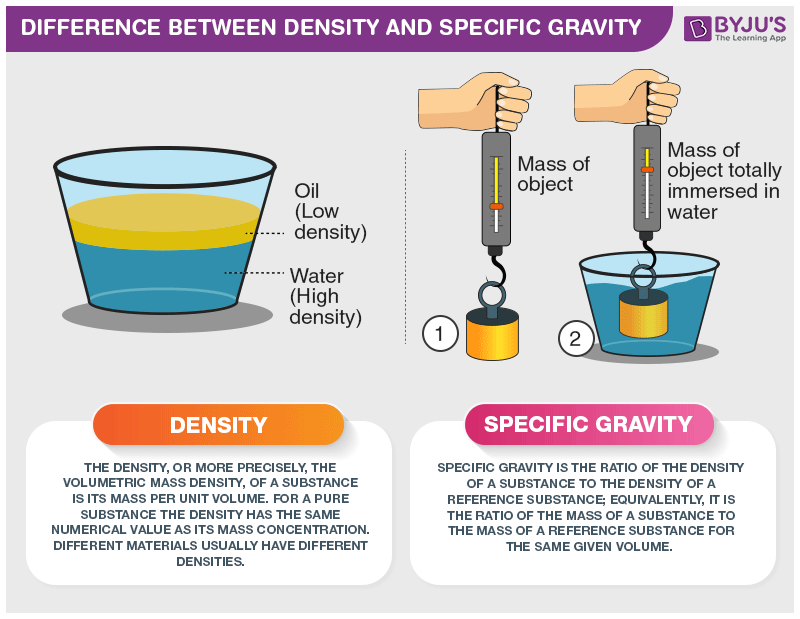# Difference Between Density and Specific Gravity

There is a noticeable difference between density and specific gravity, even though both are used to represent mass and are used to compare different substances. Density is the property of matter represented by a ratio of mass to a unit volume of matter. Specific gravity, which is also called relative density, is a measure of density with respect to the density of pure water. There are many properties of water, such as conductivity of water and many more.## Difference Between Specific Gravity and Density

If a material has a specific gravity less than 1, it will float on water.

It is represented as,

$$\begin{array}{l}specific\; gravity_{substance}=\rho _{substance}/\rho _{reference}\end{array}$$

Density, on the other hand, is represented as,

$$\begin{array}{l}\rho = mass/volume\end{array}$$

And it is represented in kilograms per cubic metre.

 Difference Between Density and Specific Gravity Density Specific Gravity (Relative Density) It is the mass of an object per unit volume. Relative density is the ratio of a substance’s weight to the weight of the same volume of water. To calculate density, we need to know the weight and volume of the substance. We can get this by dividing the density of a substance with the density of water. It is represented in absolute terms. It is represented in relative terms. Its symbol is ρ is represented in kilogram per cubic metre. It does not have an SI unit. It is used in many domestic and commercial applications. It is used in industries to measure the concentration of solutions.

These were some differences between specific gravity (relative density) and density. If you wish to find out more, download BYJU’S – The Learning App.

RELATED ARTICLES:

Test your knowledge on Density and specific gravity difference